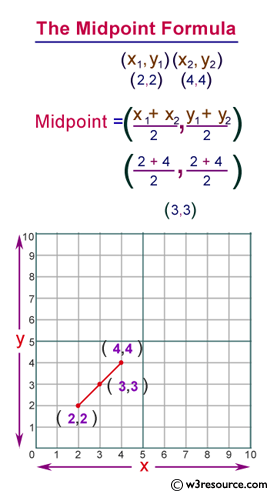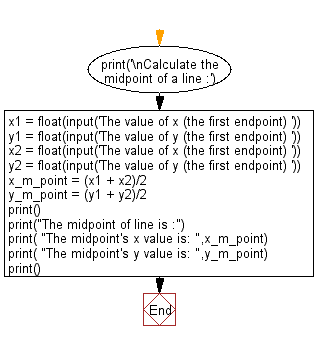﻿ Python: Calculate midpoints of a line - w3resource# Python: Calculate midpoints of a line

## Python Basic: Exercise-73 with Solution

Write a Python program to calculate midpoints of a line.

Pictorial Presentation:Sample Solution:-

Python Code:

``````print('\nCalculate the midpoint of a line :')

x1 = float(input('The value of x (the first endpoint) '))
y1 = float(input('The value of y (the first endpoint) '))

x2 = float(input('The value of x (the first endpoint) '))
y2 = float(input('The value of y (the first endpoint) '))

x_m_point = (x1 + x2)/2
y_m_point = (y1 + y2)/2
print();
print("The midpoint of line is :")
print( "The midpoint's x value is: ",x_m_point)
print( "The midpoint's y value is: ",y_m_point)
print();
```
```

Sample Output:

```Calculate the midpoint of a line :
The value of x (the first endpoint) 2
The value of y (the first endpoint) 2
The value of x (the first endpoint) 4
The value of y (the first endpoint) 4

The midpoint of line is :
The midpoint's x value is:  3.0
The midpoint's y value is:  3.0
```

Flowchart:## Visualize Python code execution:

The following tool visualize what the computer is doing step-by-step as it executes the said program:

Python Code Editor:

Have another way to solve this solution? Contribute your code (and comments) through Disqus.

What is the difficulty level of this exercise?

Test your Python skills with w3resource's quiz

﻿

## Python: Tips of the Day

Try-catch-else construct:

```try:
foo()
except Exception:
print("Exception occured")
else:
print("Exception didnt occur")
finally:
print("Always gets here")
```